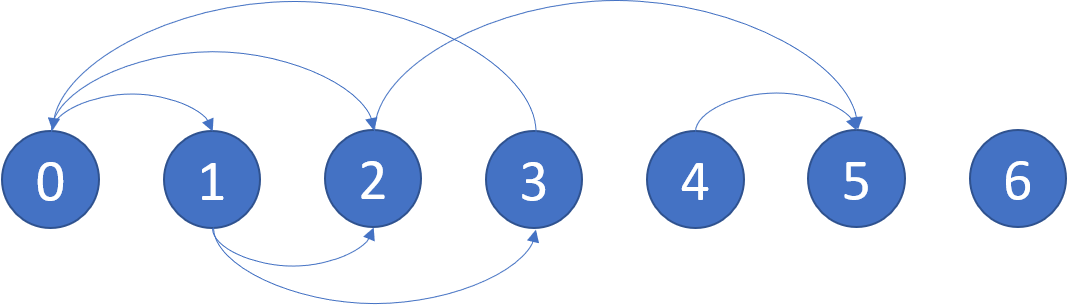# 找到最终的安全状态

## 802. 找到最终的安全状态 (Medium)```输入：graph = [[1,2],[2,3],,,,[],[]]

```

```输入：graph = [[1,2,3,4],[1,2],[3,4],[0,4],[]]

```

• `n == graph.length`
• `1 <= n <= 104`
• `0 <= graph[i].length <= n`
• `graph[i]` 按严格递增顺序排列。
• 图中可能包含自环。
• 图中边的数目在范围 `[1, 4 * 104]` 内。

### 相关话题

[深度优先搜索] [广度优先搜索] [] [拓扑排序]